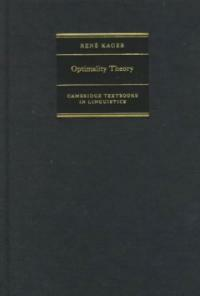> 상세정보

# 상세정보## Optimality theory (21회 대출)

자료유형
단행본
개인저자
Kager, René.
서명 / 저자사항
Optimality theory / René Kager.
발행사항
London :   Cambridge University Press,   1999.
형태사항
452 p. ; 24 cm.
총서사항
Cambridge textbooks in linguistics
ISBN
0521580196 (hbk.) 0521589800 (pbk.)
서지주기
Includes bibliographical references and indexes.
일반주제명
Optimality theory (Linguistics).
 000 00000cam u2200205 a 4500 001 000000646131 005 20151007093452 008 980803s1999 enk b 001 0 eng 010 ▼a 98039103 020 ▼a 0521580196 (hbk.) 020 ▼a 0521589800 (pbk.) 040 ▼a DLC ▼c DLC ▼d 211009 041 0 ▼a eng ▼a mul 049 ▼a OCLC ▼l 111137117 050 0 0 ▼a P158.42 ▼b .K35 1999 082 0 0 ▼a 410/.1 ▼2 23 084 ▼a 410.1 ▼2 DDCK 090 ▼a 410.1 ▼b K11o 100 1 ▼a Kager, René. 245 1 0 ▼a Optimality theory / ▼c René Kager. 260 ▼a London : ▼b Cambridge University Press, ▼c 1999. 300 ▼a 452 p. ; ▼c 24 cm. 490 1 ▼a Cambridge textbooks in linguistics 504 ▼a Includes bibliographical references and indexes. 650 0 ▼a Optimality theory (Linguistics). 830 0 ▼a Cambridge textbooks in linguistics.

### 소장정보

No. 소장처 청구기호 등록번호 도서상태 반납예정일 예약 서비스
No. 1 소장처 청구기호 410.1 K11o 등록번호 111137117 도서상태 대출가능 반납예정일 예약 서비스

### 컨텐츠정보

#### 목차

```
CONTENTS
Preface = xi
1 Conflicts in grammars = 1
1.1 Introduction goals of linguistic theory = 1
1.2 Basic concepts of OT = 3
1.3 Examples of constraint interaction = 14
1.4 The architecture of an OT grammar = 18
1.5 Interactions of markedness and faithfulness = 27
1.6 Lexicon Optimization = 32
1.7 A factorial typology of markedness and faithfulness = 34
1.8 On defining segment inventories = 43
1.9 Conclusion = 47
2 The typology of structural changes = 52
2.1 Introduction = 52
2.2 Nasal substitution and related effects = 59
2.3 The typology of N \mathopC\limts ? effects = 78
2.4 Conspiracies of nasal substitution and other processes = 83
2.5 Conclusion : a comparison with rule-based theory = 86
3 Syllable structure and economy = 91
3.1 Introduction = 91
3.2 The basic syllable typology = 92
3.3 Epenthesis and the conflict of well-formedness and faithfulness = 98
3.4 Generalized Alignment = 117
3.5 The quality of epenthetic segments = 124
3.6 Coda conditions = 130
3.7 Conclusion = 139
4 Metrical structure and parallelism = 142
4.1 Introduction = 142
4.2 Word stress : general background = 143
4.3 Case-study : rhythmic lengthening in Hixkaryana = 148
4.4 A set of metrical constraints = 161
4.5 Case-study : rhythmic syncope in Southeastern Tepehuan = 177
4.6 Conclusions = 189
5 Correspondence in reduplication = 194
5.1 Introduction = 194
5.2 Reduplicative identity : the constraints = 201
5.3 From classical templates to generalized templates = 216
5.4 From circumscription to alignment = 223
5.5 'Classical' versus OT-based prosodic morphology : conclusions = 229
5.6 Overapplication and underapplication in reduplication = 230
5.7 Summary of Correspondence Theory = 248
6 Output-to-output correspondence = 257
6.1 Introduction = 257
6.2 Identity effects in truncation = 259
6.3 Identity effects in stem-based affixation = 273
6.4 The cycle versus base-identity = 277
6.5 Output-to-output correspondence : conclusions = 293
7 Learning OT grammars = 296
7.1 Introduction = 296
7.2 Learning constraint rankings = 297
7.3 Learning the Pintupi grammar of stress = 300
7.4 The learning algorithm : discussion = 321
7.5 Learning alternations and input representations = 324
8 Extensions to syntax = 341
8.1 Introduction = 341
8.2 OT and syntax = 341
8.3 The structure of extended verbal projections in English = 353
8.4 Typological consequences = 366
8.5 Conclusions = 369
9 Residual issues = 372
9.1 Introduction = 372
9.2 Opacity = 372
9.3 Absolute ungrammaticality = 400
9.4 Free variation = 404
9.5 Positional faithfulness = 407
9.6 Underlying Representations versus allomorphy = 413
9.7 Conclusion : future perspectives = 420
References = 425
Index of languages = 445
Index of subjects = 447
Index of constraints = 451```

### 관련분야 신착자료

#### Ferdinand de Saussure : une science du langage pour une science de l'humain

Bronckart, Jean-Paul (2022)

김형민 (2022)

#### 서유럽의 언어들 : 기원, 역사, 지리

Walter, Henriette (2022)